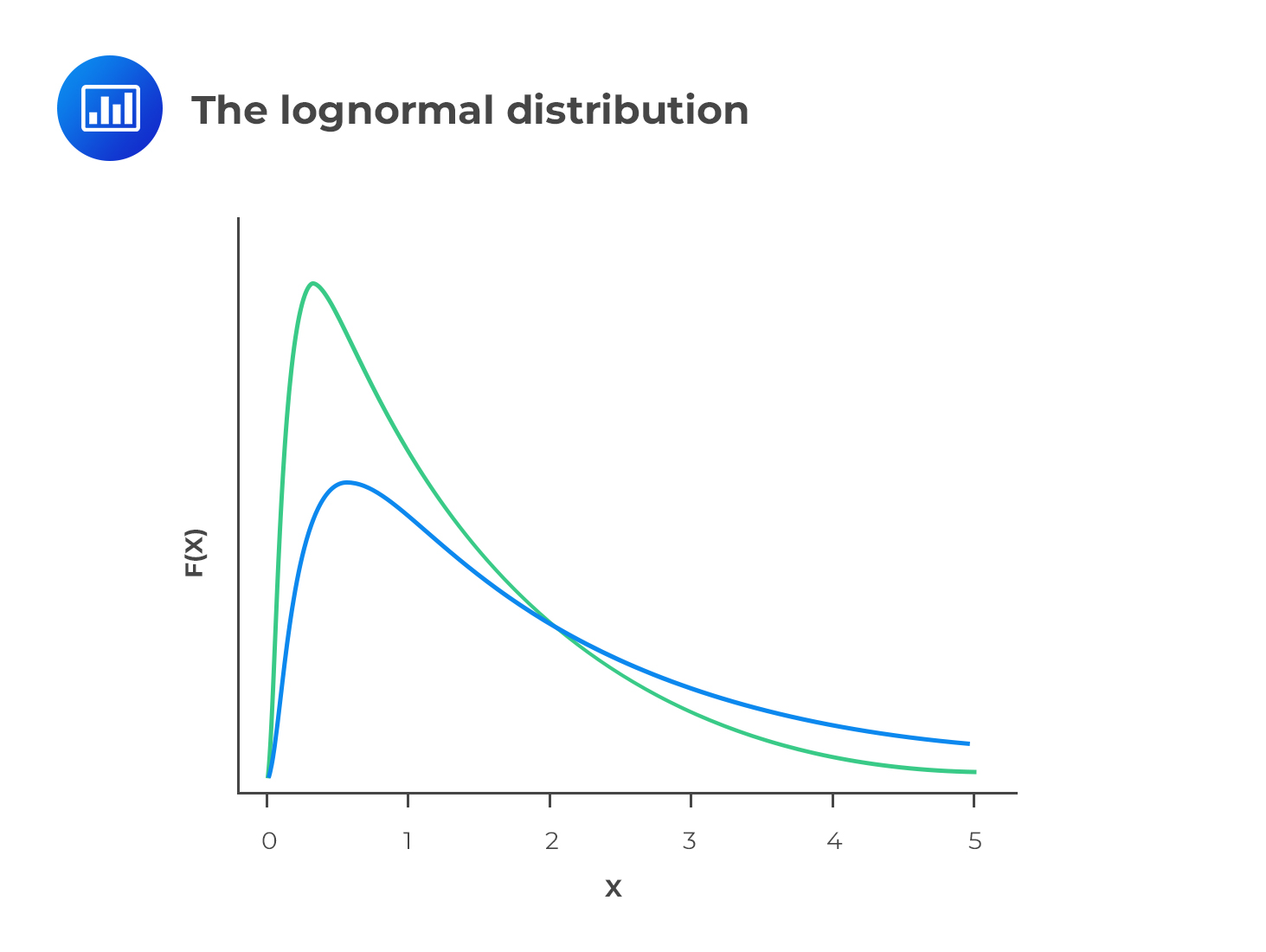# Relationship between Normal Distribution and Lognormal Distribution

A variable $$X$$ is said to have a lognormal distribution if $$Y = ln(X)$$ is normally distributed, where “ln” denotes the natural logarithm. In other words, when the logarithms of values form a normal distribution, we say that the original values have a lognormal distribution.

Let’s consider this:

$$Y=e^X$$

Where $$e$$ is the exponential constant.

If we take natural logs on both sides, $$lnY = ln\ e^X$$, which leads us to $$lnY = X$$. Therefore, if $$X$$ has a normal distribution, then $$Y$$ has a lognormal distribution.

The lognormal distribution is positively skewed, with many small values and just a few large values. Consequently, the mean is greater than the mode in most cases.## Why the Lognormal Distribution is Used to Model Stock Prices

Since the lognormal distribution is bound by zero on the lower side, it is perfect for modeling asset prices that cannot take negative values. On the other hand, the normal distribution cannot be used for the same purpose because it has a negative side.

When the returns on a stock (continuously compounded) follow a normal distribution, the stock prices follow a lognormal distribution. Note that even if returns do not follow a normal distribution, the lognormal distribution is still the most appropriate for stock prices.

The probability density function of the distribution is:

$$f\left( x \right) =\frac { 1 }{ x\sqrt { 2\pi { \sigma }^{ 2 } } } { e }^{ -\frac { { \left( lnx-\mu \right) }^{ 2 } }{ \sqrt { 2{ \sigma }^{ 2 } } } }$$

The Black-Scholes-Merton model used to price options, which we will see in-depth in level II, uses the lognormal distribution as its foundation.

### Question

Which of the following statements regarding the properties of lognormal distributions is the least accurate?

1. Lognormal distributions are skewed to the right.
2. Lognormal distributions are more often used to model asset prices than standard distributions.
3. Lognormal distributions can take negative values.

Solution

Lognormal distributions are bounded by 0 because they cannot take negative values. Since normal distributions can take negative values, and asset prices cannot be negative, lognormal distributions are more suitable for describing distributions of asset prices.

Exam tip: If you have negative values, your data isn’t lognormal.

A is incorrect. It is a true statement. Lognormal distributions are bound by zero. This implies that they do not have negative values and are thus skewed to the right.
B is incorrect. Lognormal distributions are bound by zero and thus cannot take negative values.

Shop CFA® Exam Prep

Offered by AnalystPrepLevel I
Level II
Level III
All Three Levels
Featured Shop FRM® Exam PrepFRM Part I
FRM Part II
FRM Part I & Part II
Learn with Us

Subscribe to our newsletter and keep up with the latest and greatest tips for success
Shop Actuarial Exams PrepExam P (Probability)
Exam FM (Financial Mathematics)
Exams P & FM
Shop GMAT® Exam PrepComplete CourseSergio Torrico
2021-07-23
Excelente para el FRM 2 Escribo esta revisión en español para los hispanohablantes, soy de Bolivia, y utilicé AnalystPrep para dudas y consultas sobre mi preparación para el FRM nivel 2 (lo tomé una sola vez y aprobé muy bien), siempre tuve un soporte claro, directo y rápido, el material sale rápido cuando hay cambios en el temario de GARP, y los ejercicios y exámenes son muy útiles para practicar.diana
2021-07-17
So helpful. I have been using the videos to prepare for the CFA Level II exam. The videos signpost the reading contents, explain the concepts and provide additional context for specific concepts. The fun light-hearted analogies are also a welcome break to some very dry content. I usually watch the videos before going into more in-depth reading and they are a good way to avoid being overwhelmed by the sheer volume of content when you look at the readings.Kriti Dhawan
2021-07-16
A great curriculum provider. James sir explains the concept so well that rather than memorising it, you tend to intuitively understand and absorb them. Thank you ! Grateful I saw this at the right time for my CFA prep.nikhil kumar
2021-06-28
Very well explained and gives a great insight about topics in a very short time. Glad to have found Professor Forjan's lectures.Marwan
2021-06-22
Great support throughout the course by the team, did not feel neglectedBenjamin anonymous
2021-05-10
I loved using AnalystPrep for FRM. QBank is huge, videos are great. Would recommend to a friendDaniel Glyn
2021-03-24
I have finished my FRM1 thanks to AnalystPrep. And now using AnalystPrep for my FRM2 preparation. Professor Forjan is brilliant. He gives such good explanations and analogies. And more than anything makes learning fun. A big thank you to Analystprep and Professor Forjan. 5 stars all the way!michael walshe
2021-03-18
Professor James' videos are excellent for understanding the underlying theories behind financial engineering / financial analysis. The AnalystPrep videos were better than any of the others that I searched through on YouTube for providing a clear explanation of some concepts, such as Portfolio theory, CAPM, and Arbitrage Pricing theory. Watching these cleared up many of the unclarities I had in my head. Highly recommended.